# Information, rate of generation of

(diff) ← Older revision | Latest revision (diff) | Newer revision → (diff)

A quantity characterizing the amount of information (cf. Information, amount of) emanating from an information source in unit time. The rate of generation of information of an information sourcewith discrete time generating the communication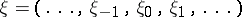, formed by a sequence of random variables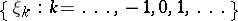taking values from some discrete set, is defined by the equation(*)

if this limit exists. Here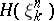is the entropy of the random variable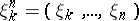. The variable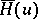, defined by (*), is also called the entropy (in a symbol) of the information source.

In certain cases one can successfully prove the existence of the limit in (*) and calculate it explicitly, e.g. in the case of stationary sources. Explicit formulas forhave been obtained for stationary Markov sources and Gaussian sources. The concept of a rate of generation of information is closely related to that of redundancy of an information source.

Ifis a stationary ergodic information source with finite number of states, then the following property of asymptotic uniform distribution (the McMillan theorem, ) holds. Let, where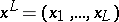are the values of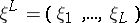in an information interval of length. For any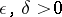, there is an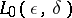such that for all,How to Cite This Entry:
Information, rate of generation of. Encyclopedia of Mathematics. URL: http://encyclopediaofmath.org/index.php?title=Information,_rate_of_generation_of&oldid=15080
This article was adapted from an original article by R.L. DobrushinV.V. Prelov (originator), which appeared in Encyclopedia of Mathematics - ISBN 1402006098. See original article